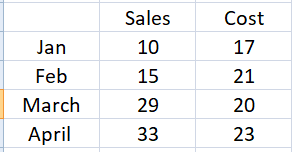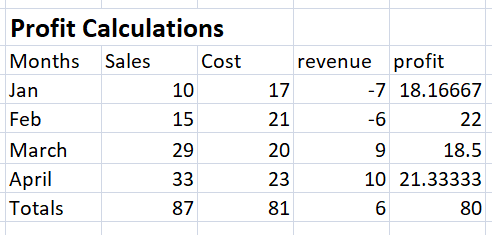Top
 Objective:  Participants will be able to understand, apply and interpret basic arithmetic and formatting in excel. Total Time: 90 Minutes Materials Required:  Computer lab with projector and screen, MS Excel installed on all the computers for students Instructor Note:  This session is the beginning of MS Excel. Based on the understanding level and pre-existing skill set of students, use demonstration as a training method and make students practice as much as possible. In this session, basic arithmetic (Summation, Subtraction, Percentages) and formatting will be covered. Opening Protocol: Greet the participants Take attendance In pairs, ask participants to share with each other how they are feeling Recap the previous session by asking 1-2 participants to share with the class, what they learnt and did in the previous sessions of Digital Literacy 5 Minutes Opening Activity: Ask students if they have ever used or seen MS Excel Tell them the basic use of excel With the help of projector, demonstrate opening a blank sheet Introduce basics of excel- Rows Columns Cell Tabs at the top 10 Minutes Activity 1: Discuss and draw a table Ask students to sit with their group members Ask them to open MS Excel in their handbook and read the instructions for Activity 1 Students will get 5 minutes to discuss and decide the table they want to create and why Ask group representatives to tell the rows and columns they would like to create and why Create the following table on the board, and then with the help of projector, demonstrate creating the same table in excel15  Minutes Activity 2: Create a table Ask students to read instructions of Activity 2 in the handbook: Create tables in excel with months as rows and Sales, Cost as columns.  Fill dummy data  Perform arithmetic  Summation , =SUM Subtraction, = sales-cost Adding percentages  Formula = revenue-cost/ revenue With the help of projector, demonstrate creating table as per the instructions  Ask students to create the same table in excel, give them 10 minutes to do this activity After 10 minutes, ask students to check each other’s excel  Randomly check some excels Demonstrate again if students found this activity difficult Outcome:25  Minutes Concept Discussion: Ask if some columns are more important than others ? Can changing the colour of certain values give it more importance ?  Introduce them to Formatting.  Take suggestions to make the table more readable  Tell them the importance of formatting in excel to make the information more relevant 10 Minutes